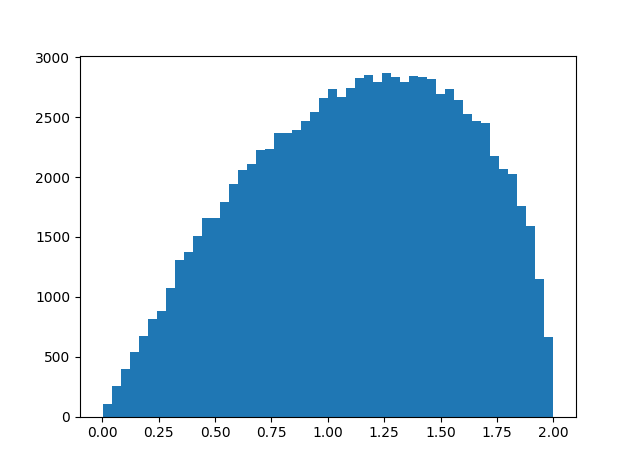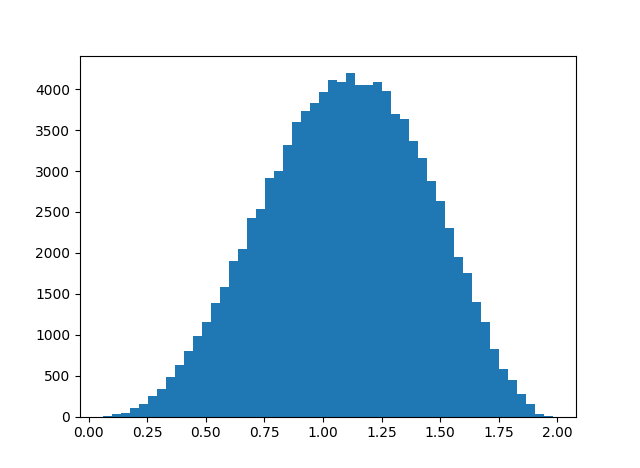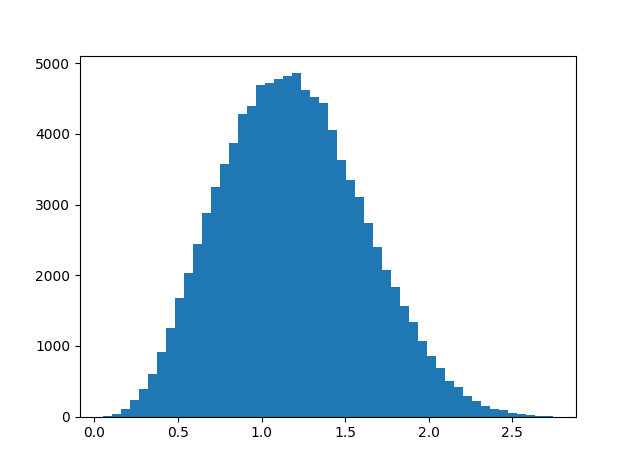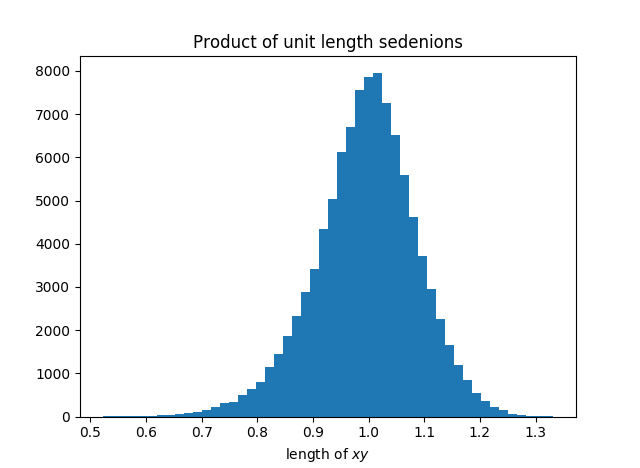# Redoing division algebra graphs with angular distance

The blog post that kicked off the recent series of posts looked at how far apart xy and yx are for quaternions. There I used the Euclidean distance, i.e. || xy – yx ||. This time I’ll look at the angle between xy and yx, and I’ll make some analogous graphs for octonions.

For vectors x and y in three dimensions, the dot product satisfieswhere θ is the angle between the two vectors. In higher dimensions, we can turn this theorem around and use it as the definition of the angle between two vectors based on their dot product.

(The plots below are jagged because they’re based on random sampling.)

Here’s the Euclidean distance between xy and yx for quaternions from the earlier post:And here’s the corresponding angular distance:The range has changed from [0, 2] to [o, π], and the distribution now shifts left instead of right.

Here’s a similar graph, looking at the angular distance between xy and yx for octonions, something I haven’t plotted before in Euclidean distance.This graph is more symmetric, which we might expect: since octonions have less algebraic structure than quaternions, we might expect the relationship between xy and yz to behave more erratically, and for the plot to look more like a normal distribution.

Finally, let’s revisit the distance between (xy)z and x(yz) for octonions. Here is the distribution of the Euclidean distance from a previous post:And here is the corresponding histogram based on angular distance.These plots are based on uniform random samples of quaternions and octonions of length 1, i.e. points from the unit spheres in 4 and 8 dimensions respectively. Quaternions and octonions have the property that the product of unit length vectors is another unit length vector, and the angle between to unit vectors is the inverse cosine of their dot product.

I thought that sedenions also had this norm property, that the product of unit length vectors has unit length. Apparently not, as I discovered by trying to take the inverse cosine of a number larger than 1. So what is the distribution of lengths that come from multiplying two sedenions of length 1? Apparently the mean is near 1, and here’s a histogram.## 4 thoughts on “Redoing division algebra graphs with angular distance”

1. “I thought that sedenions also had this norm property, that the product of unit length vectors has unit length.”

No: if they did, we’d have |ab| = |a| |b| for sedenions, so they’d be a “division algebra”, meaning that ab = 0 only if a = 0 or b = 0. But in fact division algebras are only possible in dimensions 1, 2, 4 and 8.

An algebra over the real numbers with a norm obeying |ab| = |a| |b| is called a “normed division algebra”. The real numbers, complex numbers, quaternions and octonions are the only normed division algebras.

There are uncountably many other division algebras of dimensions 4 and 8, all nonassociative. There is even a commutative but nonassociative division algebra of dimension 2! To get it, just take the complex numbers and define a new product for a and b to be the usual product of a and the complex conjugate of b.

2. Thanks. I didn’t make the connection between zero divisors and how multiplication interacts with norms, even though it’s trivial.

If I understand correctly, the sedenions are “nicely normed” in the technical sense, but not in the sense that multiplication interacts as nicely with norms as you might expect.

3. Right. I made up the term “nicely normed” and explained it on that webpage you probably read:

http://math.ucr.edu/home/baez/octonions/node5.html

A *-algebra is “nicely normed” if a+a* is a real multiple of the identity, aa* = a*a, and aa* is a strictly positive multiple of the identity when a is nonzero.

If a *-algebra is nicely normed, we can define a norm on it by |a| = sqrt(aa*) (where I’m identifying aa* with the real number you multiply the identity to get aa*). There’s no reason to expect this norm obeys

|ab| = |a| |b|

so we don’t get a normed division algebra in general, but we do get a multiplicative inverse for any nonzero a, namely a*/|a|.

Iterated Cayley-Dickson constructions starting from the real numbers give an infinite sequence of nicely normed algebras. The first one that’s not a normed division algebra is the sedenions. So, this is a great example of an algebra that’s not a normed division algebra – nor even a division algebra – but which still has a multiplicative inverse for each nonzero element.

I’m mainly mentioning this stuff because it took me some time to figure it out, and if I don’t talk about it every 5 or 10 years I’ll forget it! It’s sort of tricky stuff.

4. Sorry, that a*/|a| should be a*/|a|^2.